# Using The OPTICS Cordillera

#### 2023-01-16

In this document we give a high-level tutorial for using the functionality available in the cordillera package. The technical details are described in Rusch, Hornik & Mair (2018).

The idea of the OPTICS cordillera (OC) is to quantify the “clusteredness” that is inherent in a data matrix $$X$$ in $$R^N$$. We understand clusteredness to be a spatial property of the overall arrangement of vectors in the $$N$$-dimensional space. There exists a continuum ranging from no clusteredness to perfect clusteredness and we qunatify where on that continuum a data matrix falls. Crucially, it is not the same thing as actually having or assigning clusters, it is more of a structural property of the whole data matrix. To make that distinction clearer, we also refer to this as the “accumulation tendency” and the clusters of points as accumulation. In that it shares some similarity to measures of spatial arrangement, like Ripley’s k-function, only that we try to specifically capture clusteredness. A perhaps more familiar analogy to clusteredness would be whether the vectors in a data matrix are somehow associated or not; the specific type of association being equivalent to how clustering relates to clusteredness. Clusteredness as measured by the OC can be thought of as a summary of all the clustering information in the data matrix and in that being akin to what hierarchical clustering does, only we aggregate this into a single unidimensional measure. If a data matrix has high clusteredness then the clustering can be visually appreciated in a plot and a clustering method applied to that data matrix will typically work well.

The OC is a nonparametric measure in the sense of trying to make as little assumptions as possible. The underlying “cluster” definition is density-based and derived from Ester et al. (1996). It is also underlying the DBSCAN algorithm (Ester et al., 1996). Essentially clusters are defined as accumulations of points that are close to each other (“density-connected” on some distance metric) or as regions with a high density of points that are separated from each other by regions of low or no point density. This definition also allows for nested clusters as regions of higher density within regions of lower density. Furthermore it contains a concept of “noise” points which are points in regions where the overall density is too low for this to count as a cluster. To achieve this only two assumptions must be made: First, that a cluster comprises at least $$k$$ observations (minpts) and that the density is assessed within a maximum radius $$\epsilon$$ around each point (anything not matching that will count as noise). Due to this setup there is no need to make decisions a priori about the number of accumulations or clusters, nor about the specific shape, intracluster variance or centroid. This illustrates why the concept is appealing for measuring the “clusteredness” as opposed to a specific concrete clustering.

library(dbscan)
library(cordillera)

### OPTICS

The OPTICS algorithm (Ankerst et al., 1999) is at the heart of the OPTICS cordillera. An R native OPTICS implementation is available in the dbscan package (Hahsler et al., 2019). We also provide a rudimentary interface to the OPTICS reference impementation in ELKI (which would have to be installed for it to work). OPTICS stands for Ordering Points To Infer Clustering Structure and is essentially a hierarchical clustering version of DBSCAN. It abstracts from a concrete $$\varepsilon$$ by allowing for a maximum $$\varepsilon$$, $$\epsilon$$ up to which all $$\varepsilon$$ are taken into account (so it just needs to be “large enough”). If OPTICS would be limited to only a specific $$\varepsilon$$ (like cutting a dendrogram at a specific height) it would result in the DBSCAN clustering.

Due to this being a hierarchical clustering idea, OPTICS doesn’t give a concrete clustering but orders the points based on a quantity derived from $$k$$ and $$\epsilon$$ called “reachability”. This ordering is quite complex and happens algorithmically, so it can’t be expressed in close form. What results is the tuple of ordering of the points/row vectors $$x_i$$ in $$X$$, $$R(X)$$, together with the associated reachability $$r_i$$ for $$x_i$$. This tuple contains the complete clustering structure within $$X$$ up to the maximum radius $$\epsilon$$ given an accumulation must comparise at least $$k$$ points. It holds that points that are subsequent in the ordering and have relatively low reachability belong to the same accumulation, whereas points that are far away from each other in the ordering or subsequent points with relatively high reachabilility belong to different accumulations. Every high reachability signals the beginning of a new accumulation.

This can be plotted with the ordering of the $$x_i$$ on the $$x$$-axis and their associated reachability $$r_i$$ on the $$y$$-axis. This plot is coined the “reachability plot” and is the visual expression of the complete clustering structure within $$X$$ up to the maximum radius $$\epsilon$$ given an accumulation must comparise at least $$k$$ points. The reachability plot is similar to a dendrogram in that it can be cut at some level $$\varepsilon \leq \epsilon$$ to yield a concrete clustering (the corresponding DBSCAN clustering for $$k$$ and $$\varepsilon$$).

To illustrate let’s first create a strange cluster situation in 2D.

library(clusterSim)
#three spherical clusters with different variance with n=66 each
n<-198
set.seed(1)
x <- cbind(
x = c(runif(1, -2, 5) + rnorm(n/3, sd=0.2), runif(1, -2, 5) + rnorm(n/3, sd=0.3), runif(1, -2, 5) + rnorm(n/3, sd=0.05)),
y = c(runif(1, -3, 5) + rnorm(n/3, sd=0.2), runif(1, -3, 5) + rnorm(n/3, sd=0.3), runif(1, 0, 4) + rnorm(n/3, sd=0.05))
)
cl<-c(rep(1:3,each=n/3)) #the clusters
#two worm clusters of size 100 each
set.seed(1)
sw<-shapes.worms(100,shape1x1=-2,shape1x2=0.5,shape2x1=-0.3,shape2x2=3)
#noise
set.seed(5)
ns<-cbind(runif(10,-2,5),runif(10,-2,5))
x<-rbind(x,sw$data,ns) #x<-rbind(x,sw$data)
cl<-c(cl,sw$cluster+3,rep(8,10)) #cl<-c(cl,sw$cluster+3)

plot(x, col=cl,pch=19,xlim=c(-3,5),ylim=c(-3,6))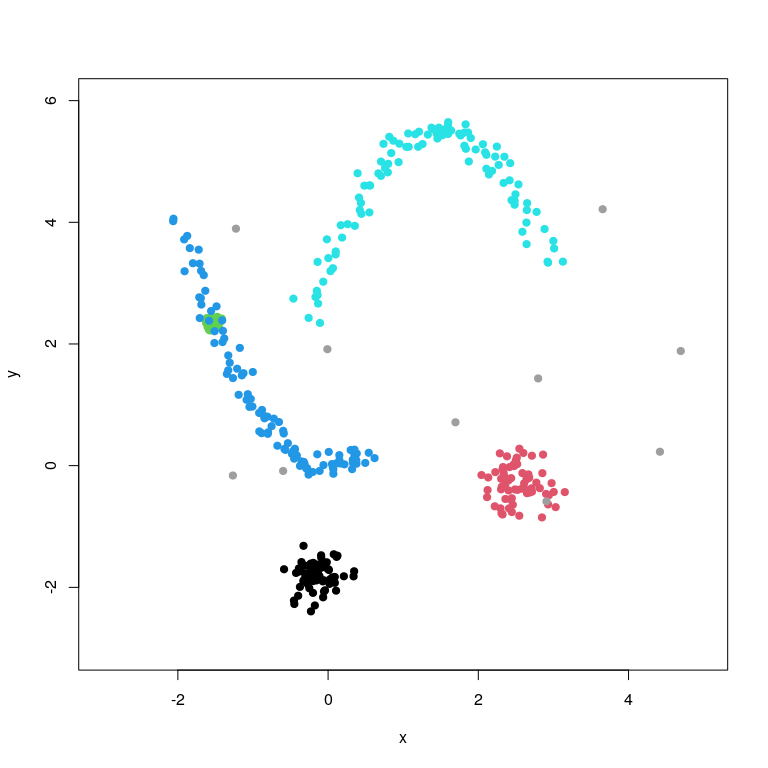We see that there are 5 clusters, two are the worms, three are spherical with different variance. The green spherical cluster is nested within the blue worm. Additionally, we have 10 noise points (in grey).

The clustering structure is clear when looking at the plot: The black, red, turquoise, green and blue clusters have a higher density of points than the surrounding area. All clusters but the green cluster are separated by regions of low or no point density. Low density because there are some noise points. The green cluster is a high density region within the blue cluster. This situation is extremely difficult to disentangle for a cluster algorithm that uses centroids, assumes constant variances or spherical shapes. Yet, we see that is quite clustered as an overall plot.

OPTICS can help us with characterizing the situation. Let’s run OPTICS on this, with the minimum number of points comprising a cluster to be $$k=22$$ and a large $$\epsilon=10$$.

ores<-optics(x,minPts=22,eps=10)
print(ores)
#> OPTICS ordering/clustering for 408 objects.
#> Parameters: minPts = 22, eps = 10, eps_cl = NA, xi = NA
#> Available fields: order, reachdist, coredist, predecessor, minPts, eps,
#>                   eps_cl, xi

The OPTICS ordering is available as $order and the reachabilities via $reachdist. We can plot those and color the plot based on the known clustering.

plot(ores,col=cl[ores$order])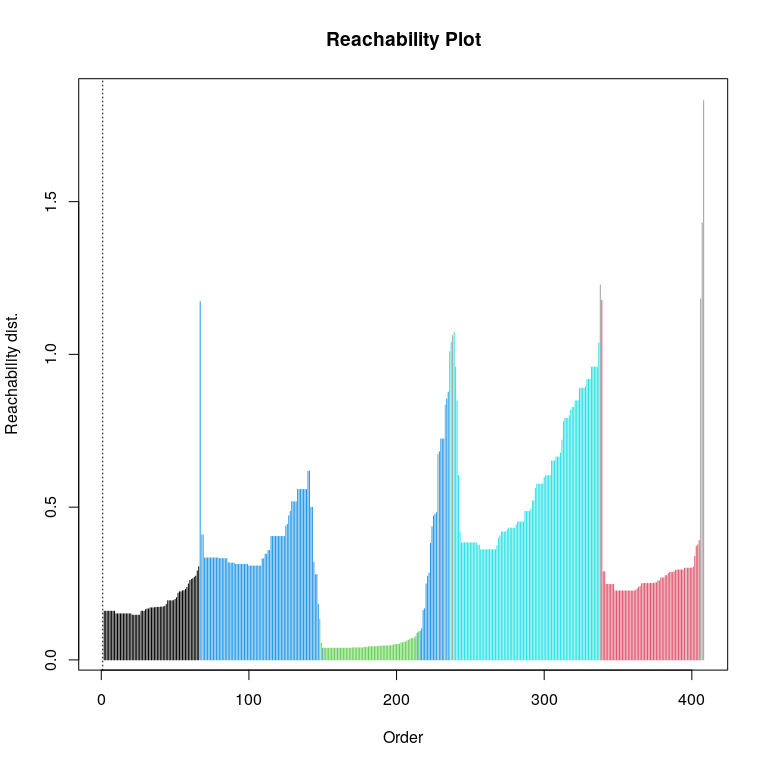The interpretation is now this: Each “valley” in the plot stands for a cluster and each cluster is separated by a peak. Valleys after low peaks are typically nested clusters. The deeper the valley, denser the cluster and the higher the peak, the more separated the clusters. From left to right we see that, the first valley is the black cluster, and then we have a peak signalling the start of the next cluster (the blue one). Then there is a peak signalling a new cluster (the green cluster) but that peak is relatively small compared to the other peaks, so we can assume that the green cluster is nested within the blue cluster (although that is certainly not conclusive here, it could also be that the green cluster is just close to the blue cluster). The next peak is signalling the turqoise cluster and then we have the red cluster after the next peak. To the topmost right are a number of high reachabilities, which would stand for noise. Note that some of the noise points are classified to be withing a cluster as they are in the density region of some of our clusters. It is of course easy to make the connections with the color coding and interpreting that without the color codes is much harder but we already see a pattern when compared to the 2D plot: We chose a $$k$$ that elicited that we have 5 clusters, that the densest cluster is the green one, followed by black, red and then the two worms. So generally we can say that 1) the deeper a valley the clearer the clustering 2) the more valleys we have the clearer the clustering and 3) the higher the peaks are the clearer the clustering. Compare this to the following situation where we moved the turqoise cluster closer to the blue one together, shifted the red and black clusters closer to the blue one and increased the variance of the red and back clusters. x2<-x x2[which(cl==5),1]<-x2[which(cl==5),1]-0.5 x2[which(cl==5),2]<-x2[which(cl==5),2]-2 x2[which(cl==1),1]<-x2[which(cl==1),1]*1.5 x2[which(cl==1),2]<-(x2[which(cl==1),2]+1.2)*1.5 x2[which(cl==2),1]<-(x2[which(cl==2),1]-1.5)*1.5 x2[which(cl==2),2]<-x2[which(cl==2),2]*1.5 plot(x2, col=cl,pch=19,xlim=c(-3,5),ylim=c(-3,6))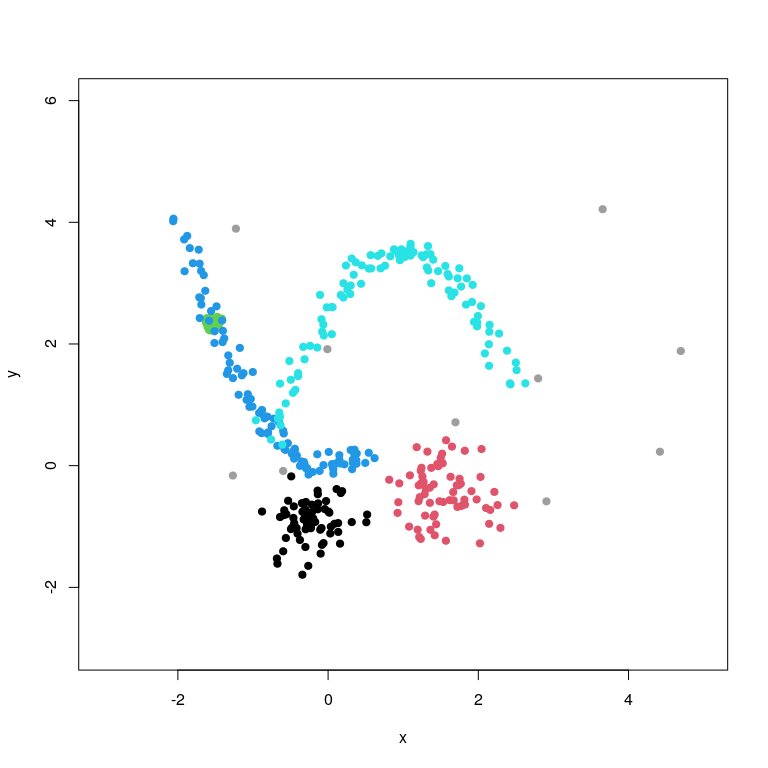This plot looks less clearly structured (clustered) than the one from before which is easiest to be seen without the coloring par(mfrow=c(1,2)) plot(x,pch=19, xlim=c(-3,5),ylim=c(-3,6)) plot(x2,pch=19, xlim=c(-3,5),ylim=c(-3,6)) par(mfrow=c(1,1))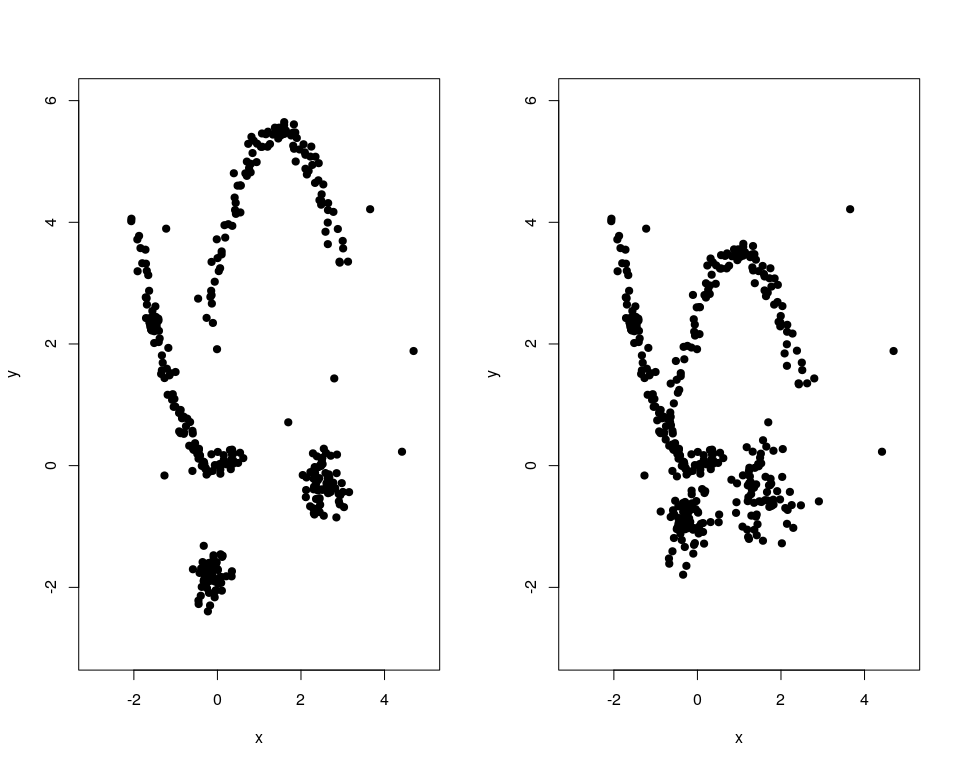Let’s look at what OPTICS tells us about the less clustered situation ores2<-optics(x2,minPts=22,eps=10) plot(ores2,col=cl[ores2$order])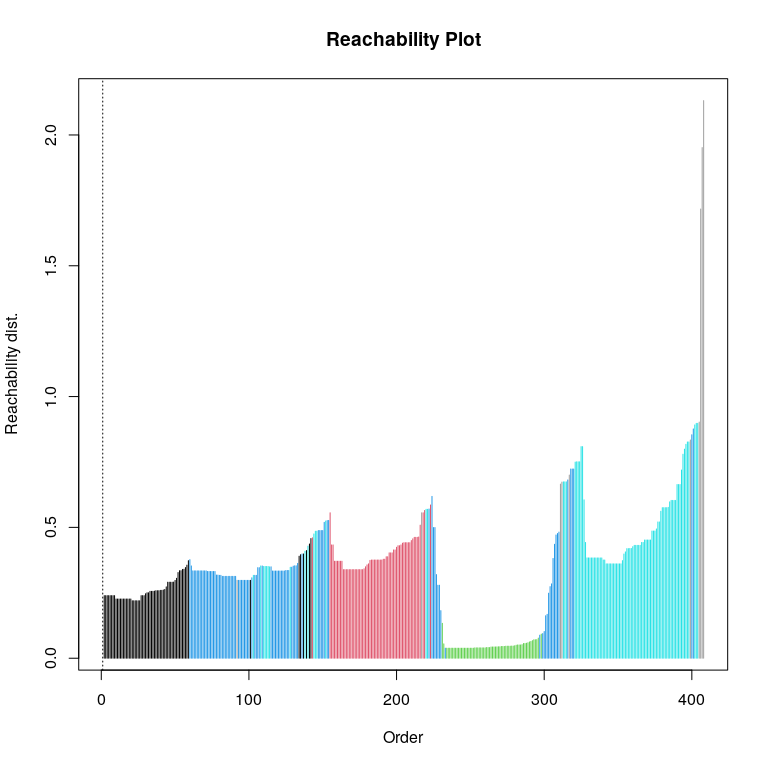We still see that OPTICS does a fairly good job in identifying the number of clusters but notice that the valleys are relatively less deep for black and red and that the peaks are much smaller compared to the first.

Now let’s have turqoise, black and red basically merged.

x3<-x
x3[which(cl==5),1]<-x3[which(cl==5),1]-1
x3[which(cl==5),2]<-x3[which(cl==5),2]-5
x3[which(cl==1),1]<-x3[which(cl==1),1]*2
x3[which(cl==1),2]<-(x3[which(cl==1),2]+1.5)*2
x3[which(cl==2),1]<-(x3[which(cl==2),1]-1.8)*2
x3[which(cl==2),2]<-x3[which(cl==2),2]*2
plot(x3, col=cl,pch=19,xlim=c(-3,5),ylim=c(-3,6))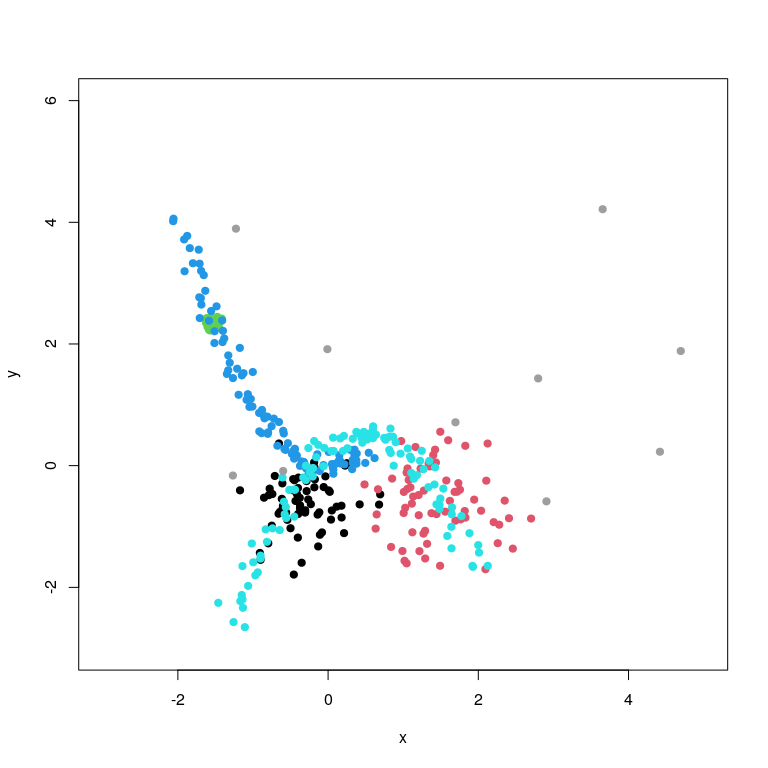This plot looks even much less clearly structured (clustered) than the ones from before. This is now just a weird-shaped blob and looks like there’s not really any clustering going on. Again this is easiest to be seen without the coloring.

par(mfrow=c(1,3))
plot(x,pch=19, xlim=c(-3,5),ylim=c(-3,6))
plot(x2,pch=19, xlim=c(-3,5),ylim=c(-3,6))
plot(x3,pch=19, xlim=c(-3,5),ylim=c(-3,6))
par(mfrow=c(1,1))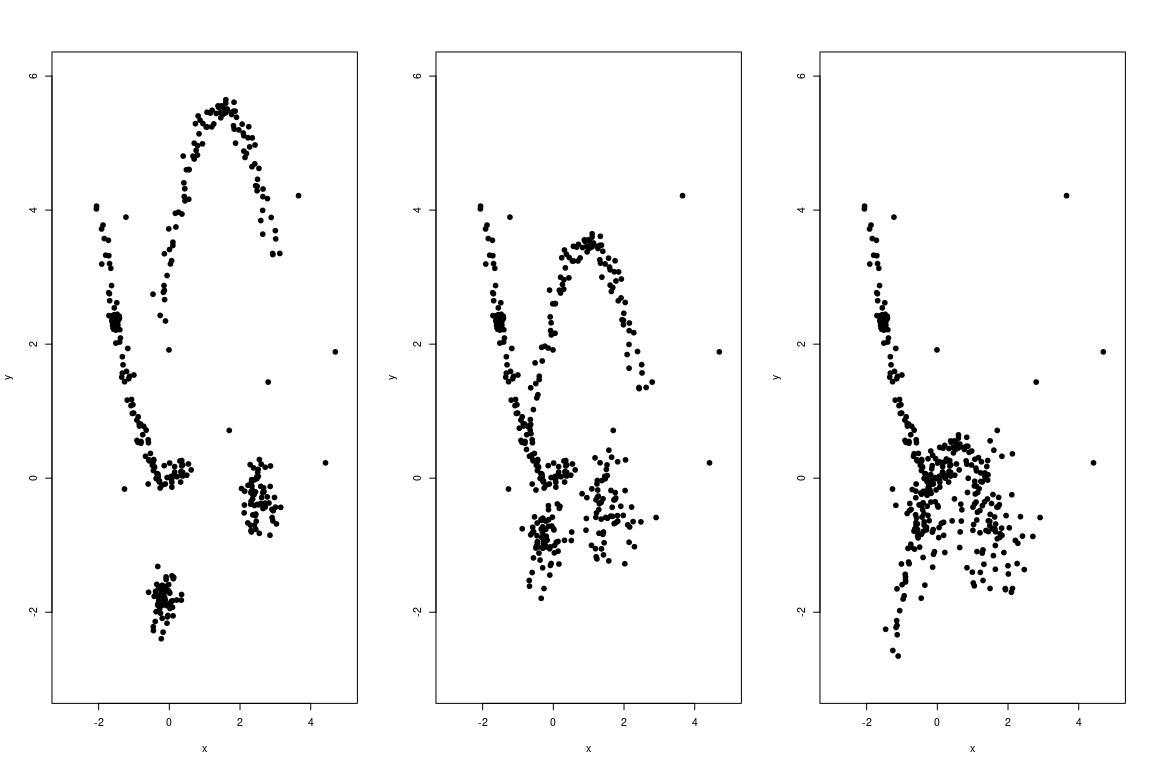Let’s look at what OPTICS tells us about the least clustered situation

ores3<-optics(x3,minPts=22,eps=10)
plot(ores3,col=cl[ores3$order])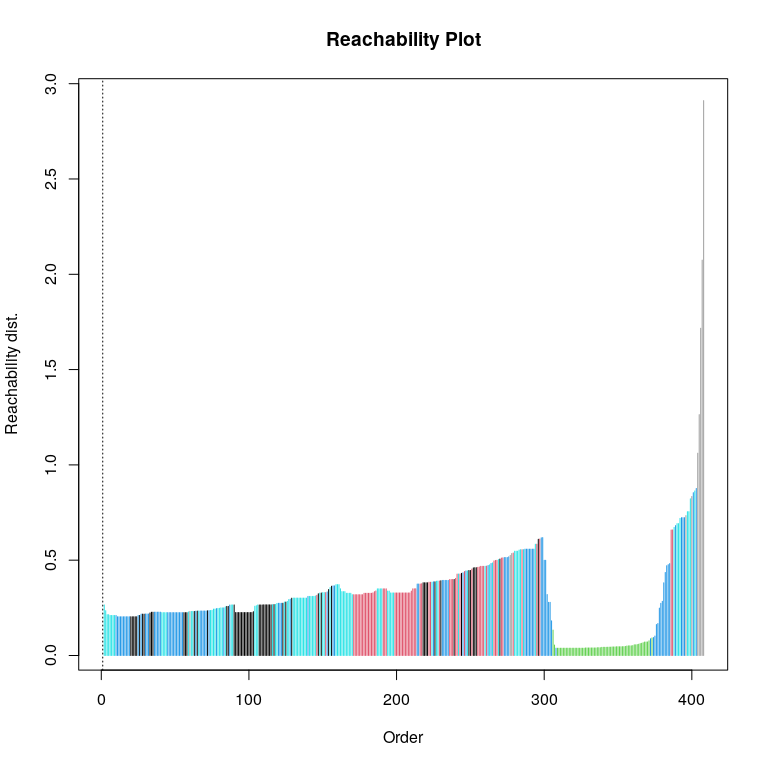It can now only identify the dense green cluster and the rest is basically just a blurred mass. Note that the peaks and the valleys are not very different or that the difference of subsequent reachabilities over the ordering is not very large (apart for the green cluster). Let’s look at the three reachability plots next to each other (note that the highest reachability is largest for the most unclustered situation here, which will be a consideration later when we talk about robustness) par(mfrow=c(1,3)) plot(ores,col=cl[ores$order],ylim=c(0,2))
plot(ores2,col=cl[ores2$order],ylim=c(0,2)) plot(ores3,col=cl[ores3$order],ylim=c(0,2))
par(mfrow=c(1,1))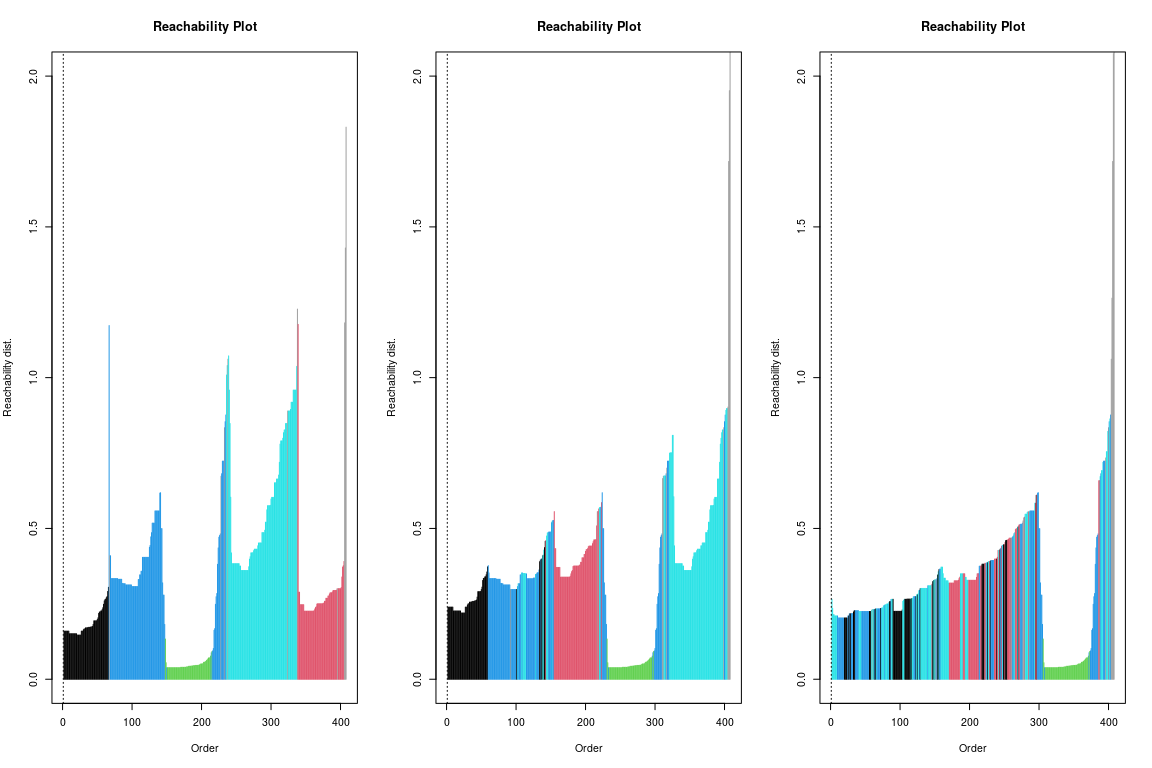So, if we agreee that the first situation is the most clustered, then the second and that one more than the third, we see that in OPTICS this translates to less “up and down” of the reachability plot for the less clustered situations, or less “raggedness” of the reachability plot.

### The OPTICS Cordillera

This observation is now at the starting point for the OPTICS Cordillera. Say, we don’t want to look at a reachability plot every time, but want one number that tells us how clustered a plot/data matrix is. We saw that in a more clustered situation, the “up and down” of the reachability plot in the sense of higher peaks and deeper valleys and bigger differences between the two, and the more clusters we have (so more peaks and more valleys), would give us a indication of the clusteredness in the data matrix.

What the OPTICS cordillera now does is precisely that: It measures the raggedness of the reachability plot by taking the norm of the subsequent reachability differences over the OPTICS ordering. The larger norm is, the more peaks, the more valleys we have, the deeper the valleys, the higher the peaks are, in short the more ragged the reachability plot is. And more of that means more clusteredness. This is where the name “cordillera” comes from.

Let’s calculate the OPTICS Cordillera for these situations with the minpts=20, epsilon=10 as before and a maximum winsorization distance of dmax=1.5. This last setting is for robustness so reachabilities larger than 1.5 get set to 1.5 (when looking at the reachability plots above, we see that the highest reachabilities are larger for the situations with less clusteredness because the cluster is much more spread out, see below for more). When comparing the OC over different $$X$$, dmax should be fixed to be the same for the OC to be comparable.

cres1<-cordillera(x,minpts=22,epsilon=10,dmax=1.5,scale=FALSE)
summary(cres1)
#>
#>   OPTICS Cordillera values with minpts= 22 and epsilon= 10
#>
#>    Raw OC: 2.232495
#>    Normalization: 83.25
#>    Normed OC: 0.24468
cres2<-cordillera(x2,minpts=22,epsilon=10,dmax=1.5,scale=FALSE)
summary(cres2)
#>
#>   OPTICS Cordillera values with minpts= 22 and epsilon= 10
#>
#>    Raw OC: 1.484021
#>    Normalization: 83.25
#>    Normed OC: 0.1626477
cres3<-cordillera(x3,minpts=22,epsilon=10,dmax=1.5,scale=FALSE)
summary(cres3)
#>
#>   OPTICS Cordillera values with minpts= 22 and epsilon= 10
#>
#>    Raw OC: 1.339798
#>    Normalization: 83.25
#>    Normed OC: 0.146841

We see that both the Raw OC (the length of the “up and down”) and the normalized version normed OC (see below) are highest for the first situation, then for the second, then for the third - exactly as we saw it in the plots above. So the OC is doing what it should.

The OC can also be visualized and is proportional to the fat black enveloping line in the reachability plot (note the effect of dmax which now winsorizes the largest, outlying reachabilities).

par(mfrow=c(1,3))
plot(cres1)
plot(cres2)
plot(cres3)
par(mfrow=c(1,1))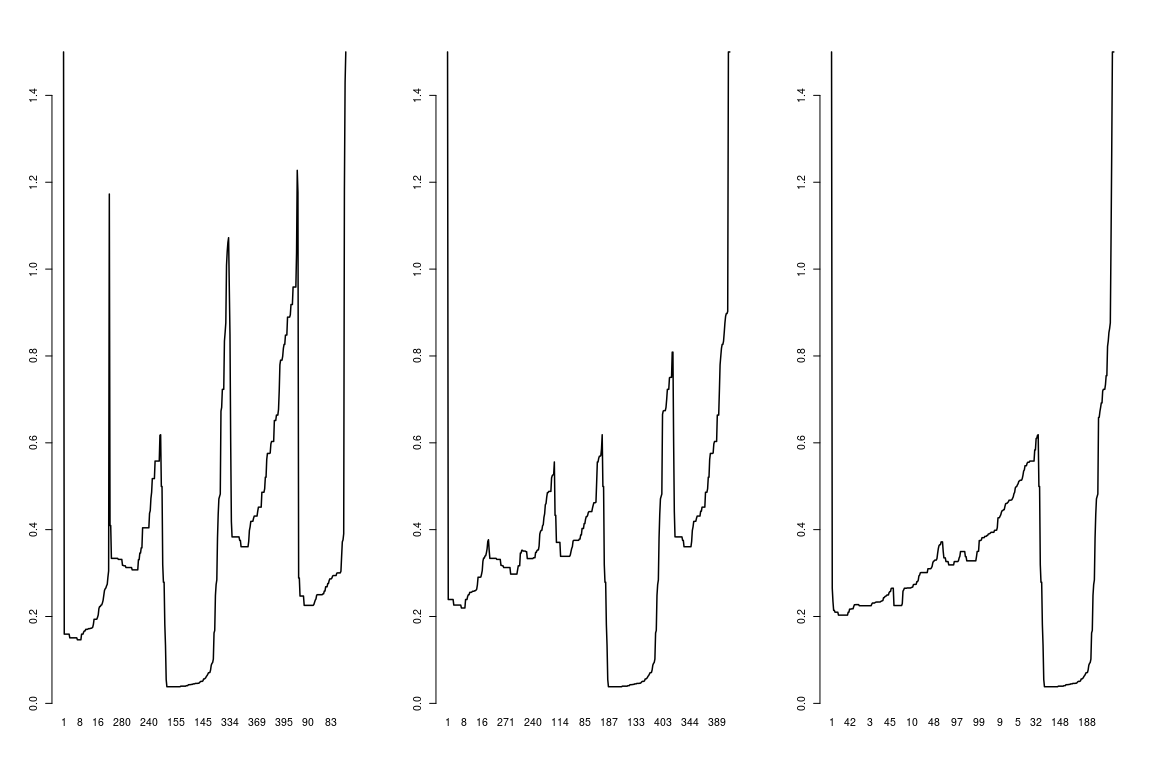We can clearly see that the length of the first black line is the longest (just imagine walking this in the Alps!).

## Normalizing the OPTICS Cordillera

The raw OC depends on the scale of the reachabilities, the number of points overall $$n$$ and also on the $$k$$ (minpts for which it holds that the lower it is the higher the raw OC typically becomes). If these are constant for two analyses with the OC, the raw values can be interpreted relatively to each other, but theoretically the scale is non-negative and unbounded ($$OC(X) \in [0,\infty)$$). The raw OC is basically the norm (length) of the up and down between the peaks and the lowest points in each cluster valley. Therefore there is a tendency that the lower minpts is (possibly deeper valleys) and the higher $$n$$ is (more possible up and downs) and the higher $$d_{max}$$ is (higher possible peaks), the higher the raw OC value becomes.

Rusch et al. (2018) derive upper and lower bounds for the raw OC for given $$n$$, $$k$$ and $$d_{max}$$ (as the maximum allowed reachability). This normalization will make the OC to lie between 0 for the least possible clusteredness (all points are equidistant to their nearest neighbour) and 1 (where we have exactly $$n/k$$ clusters, in each cluster the $$k$$ points coincide perfectly and all clusters are exactly at a distance of $$d_{max}$$ to the nearest other cluster). The normalization allows for a kind of absolute interpretation of the OC as the percent of how much clusteredness is attained relative to the most clustered arrangement possible and the normlaized value is returned as normed OC. Due to how the normalization works there is a tendency that the lower minpts, the higher $$n$$ is and the more noise points there are, the more difficult it is to realize a high normed OC.

Both the raw and the normed values are returned by the print or summary methods. The summary also gives the normalization factor.

cres1
#>      raw   normed
#> 2.232495 0.244680
summary(cres1)
#>
#>   OPTICS Cordillera values with minpts= 22 and epsilon= 10
#>
#>    Raw OC: 2.232495
#>    Normalization: 83.25
#>    Normed OC: 0.24468

## Interpreting and comparing OC values

The raw OC is basically the norm (length) of the up and down between the peaks and the lowest points in each cluster valley. Therefore there is a tendency that the lower minpts is (possibly deeper valleys) and the higher $$n$$ is (more possible up and downs) and the higher $$d_{max}$$ is (higher possible peaks), the higher the raw OC value becomes. For a single result it is therefore difficult to interpret it in terms of its magnitude due to it capturing a number of aspects such as cluster separation, cluster compactness and number of clusters simultaenously. The normed OC should then be used as it allows for an absolute interpretation as the ratio of how much clusteredness is attained relative to the most clustered arrangement possible given $$k$$, $$n$$ and $$d_{max}$$, or as percent of that if multiplied with 100.

For comparison of different representations of the same data or different data sets with equal and fixed $$k$$, $$n$$, $$d_{max}$$, the raw OC is not dimensionless and can in principle be interpreted in absolute terms between representations of the same data (see above). However, again due to the OC capturing a number of aspects such as cluster separation, cluster compactness and number of clusters simultaenously, it is difficult to say which of these is now the driving factor for the raw OC and we think it is then best to interpret it as a rank (so more raw OC is more clustered, but not exactly how much more). In such a case the normed OC can be interpreted as absolute in terms of its magnitude as it has the same normalization constant for different representations. This allows to add the interpretation of how much of the maximum possible clusteredness is achieved and therefore the values can be interpreted as differences too, so say one representation of a data set with the same $$n$$, $$k$$, $$d_{max}$$ achieves $$5\%$$ more clusteredness compared to another representation of the same data set.

For general situations of representation/data sets with different $$n$$, $$k$$ or $$d_{max}$$ for the OC (e.g., different analyses with different parameters, or different data sets) we need to point out that the OC values are typically not directly comparable. In such a situation shoudl the same $$n$$, $$k$$ or $$d_{max}$$ be used for the OC, however, both the raw (again best as a ranking) and the normed value (in the absolute) can be compared. In practice we mostly want to compare different representations of the same data, so often $$n$$ and $$k$$ are fixed anyway, which usually means we just need to fix $$d_{max}$$ for the comparison to be admissable.

The normed OC is further also comparable as a goodness-of-clusteredness statistic between different $$n$$, $$k$$ and $$d_{max}$$ if we want to relatively compare how much more of the most possible clusteredness given the setup is attained for different representations or data sets. This can mean that a situation with a higher raw OC can have less goodness-of-clusteredness if it is further away from the maximum possible clusteredness achievable, which would mean it is relatively less clustered compared to its possible maximum when compared to another.

To sum up we recommend to use the following OC values for different situations

Comparison OC value
Single data set with itself (goodness-of-clusteredness) normed OC (absolute)
Different representations of the same data with the same $$k$$, $$d_{max}$$ raw OC (as ranking), normed OC (absolute)
Different data with the same $$n$$, $$k$$, $$d_{max}$$ raw OC (as ranking), normed OC (absolute)
Different representations of the same data with different $$n$$, $$k$$, $$d_{max}$$ relative to their individual maximum clusteredness normed OC (relative)
Different data with different $$n$$, $$k$$, $$d_{max}$$ relative to their individual maximum clusteredness normed OC (relative)
Different representations/data with different $$n$$, $$k$$, $$d_{max}$$ not comparable by OC

### Interpretation examples

Let’s look again at the first example. Here we have a single data set that we want to assess for its clusteredness.

summary(cres1)
#>
#>   OPTICS Cordillera values with minpts= 22 and epsilon= 10
#>
#>    Raw OC: 2.232495
#>    Normalization: 83.25
#>    Normed OC: 0.24468

We would use the normed OC. For dmax=1.5, we achieve about $$25\%$$ of the maximum clusteredness (which would be if we had $$20$$ clusters with 22 points each that exactly coincide and each cluster was at a reachability of 1.5 form the neighbouring cluster).

Let’s now compare two representations of the same data once in the original and once where we press the y column together (rescaled to a 10th; not that this would make any sense)

rep2<-x
rep2[,2]<-rep2[,2]/10
par(mfrow=c(1,2))
plot(x,col=cl,asp=1,ylim=c(-3,6),xlim=c(-3,6))
plot(rep2,col=cl,asp=1,ylim=c(-3,6),xlim=c(-3,6))
cres1a<-cordillera(rep2,minpts=22,epsilon=10,dmax=1.5,scale=FALSE)
summary(cres1a)
#>
#>   OPTICS Cordillera values with minpts= 22 and epsilon= 10
#>
#>    Raw OC: 1.118171
#>    Normalization: 83.25
#>    Normed OC: 0.1225508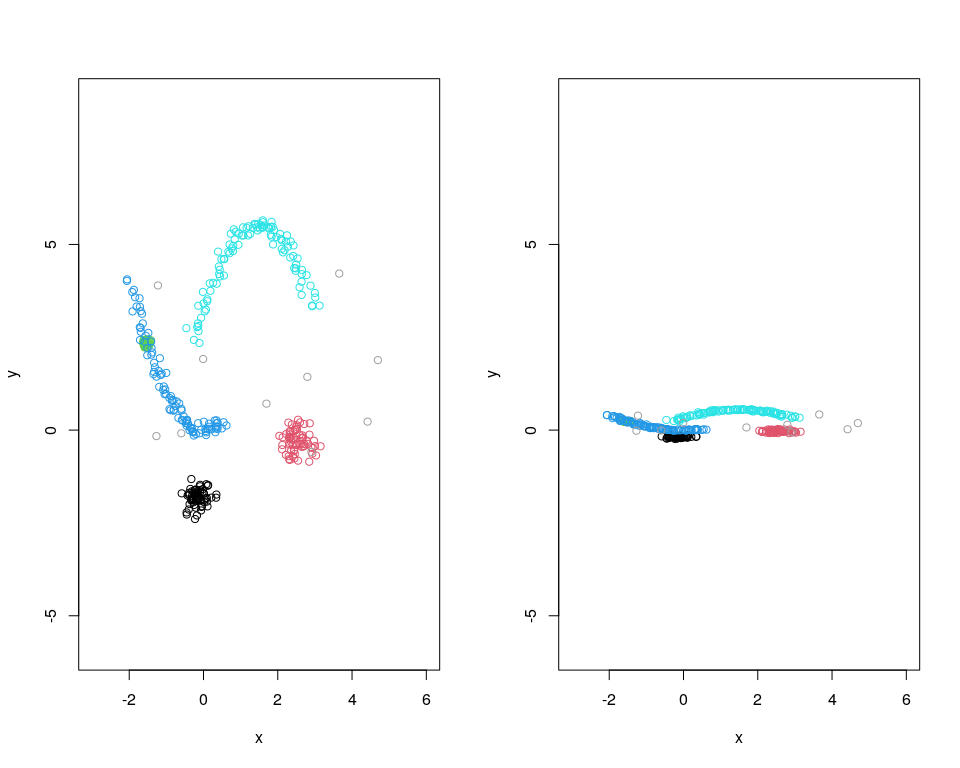We see that there is lower clusteredness in the second representations ($$12\%$$ of achievable).

### Customizing the OPTICS Cordillera

The OPTICS Cordillera can be customized with a number of parameters to allow for explore clusteredness in different settings and under different conditions.

To show these effects, we use the following toy data set with a compact cluster of 4 points where two points are denser (nested cluster of 2 points) (Cluster 1 and Cluster 1a nested within), a less compact cluster with three points (Cluster 2), a third cluster with three points of which 2 points are closer (nested cluster) (Cluster 3 and Cluster 3a nested within) and an outlier.

x<-cbind(c(3.7,-4.1,-4.3,-4.6,-4.3,3.2,6.1,4.3,-0.5,-2,-1.7),c(-7,-0.15,-0.1,1.1,-1.2,0.4,1.2,2.5,4.7,6,6.5))
cl<-c("grey",rep("black",4),rep("red",3),rep("green",3))
row.names(x)<-1:dim(x)
plot(x,type="n")
text(x,col=cl,labels=row.names(x),asp=1)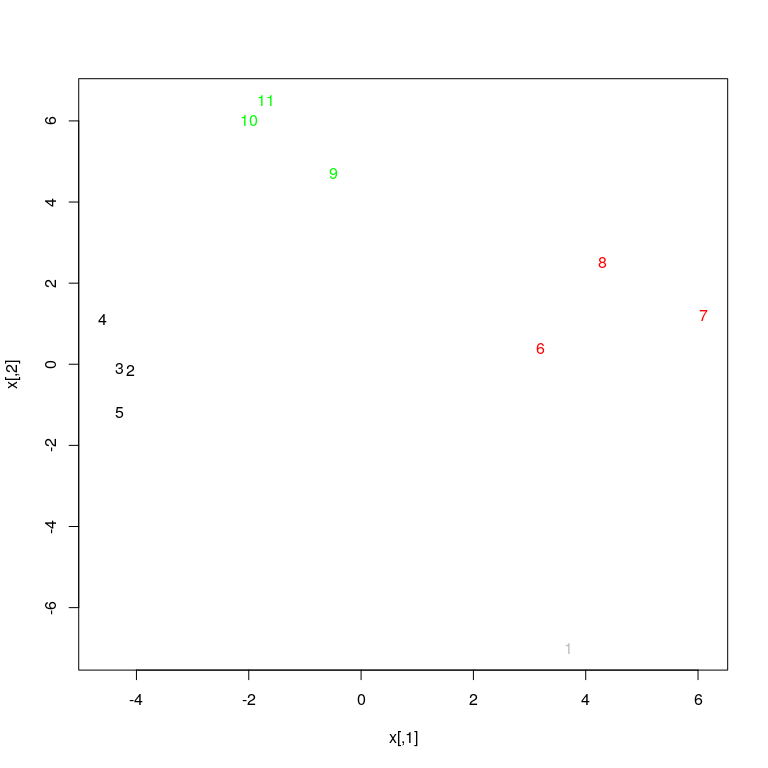minpts
The most important parameter is minpts and is a parameter for OPTICS (see there for more). It gives the minimum number of points that must make a cluster. It must be at least 2. This will influence how the clusteredness is assessed, for example if we have many 2 point clusters that are well separated and set minpts=2, OC will be high, but if we set it higher, say minpts=3, clusteredness will assessed to be lower because the 2 point clusters are no longer seen as clusters.
To illustrate for the above data, we have two three point clusters and one 4 point cluster. So if we say
ores3<-cordillera(x,minpts=3,dmax=6,scale=FALSE)
summary(ores3)
#>
#>   OPTICS Cordillera values with minpts= 3 and epsilon= 29.07989
#>
#>    Raw OC: 7.438504
#>    Normalization: 252
#>    Normed OC: 0.4685817
plot(ores3)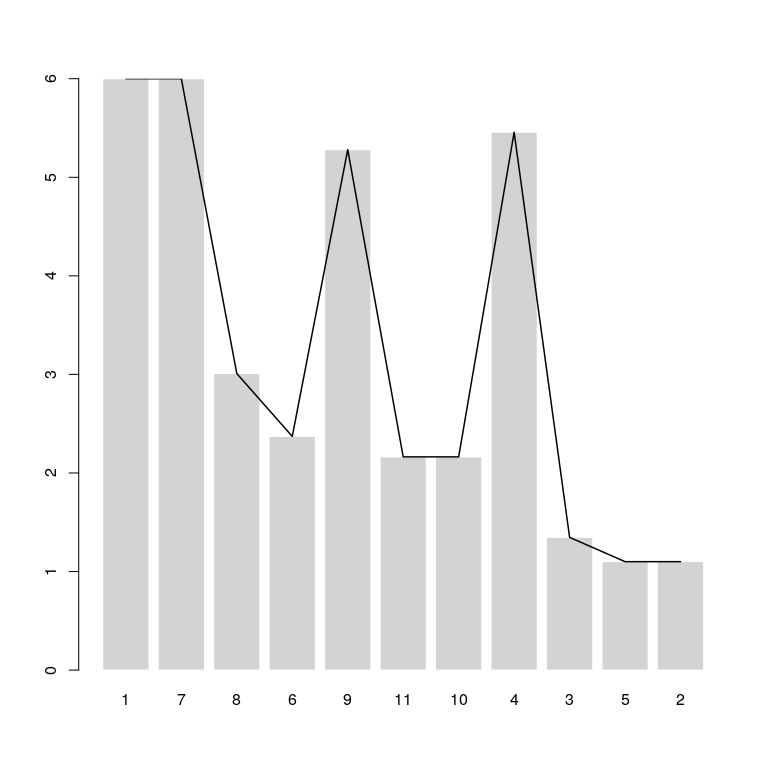we get a relatively high OC value, as all the clusters are found and the clusters have at least three objects. Compare this to setting minpts=4

ores3a<-cordillera(x,minpts=4,dmax=6,scale=FALSE)
summary(ores3a)
#>
#>   OPTICS Cordillera values with minpts= 4 and epsilon= 29.07989
#>
#>    Raw OC: 3.400002
#>    Normalization: 180
#>    Normed OC: 0.2534212
plot(ores3a)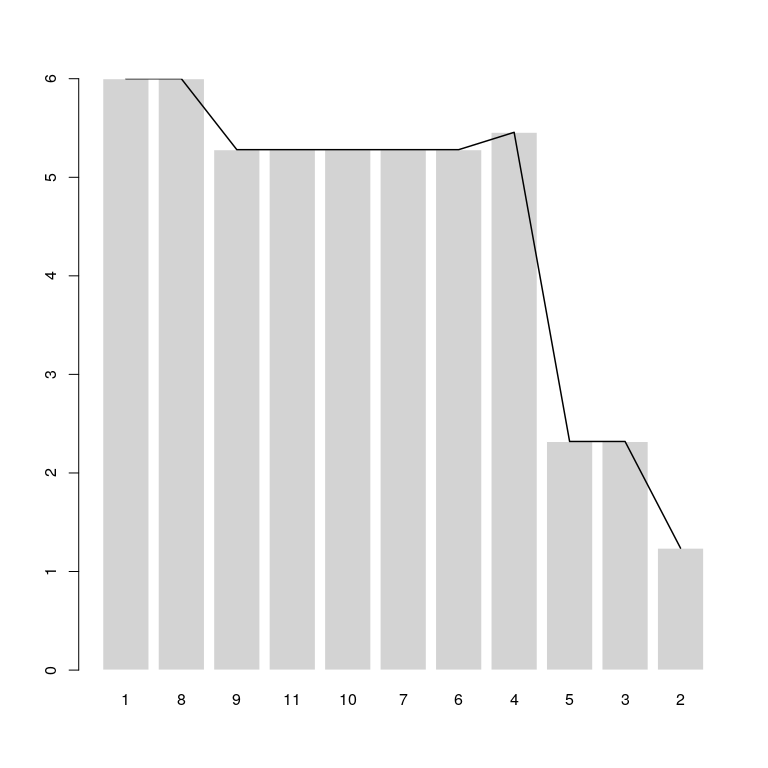which is much lower because the three point clusters no longer count as their own clusters and the reachabilities get extended between Cluster 2 and Cluster 3 so we don’t really have any peaks. The points from 6 to 11 are basically now seen as a single cluster with very low density of at least four points; the only cluster really identified is the 4 point cluster (Cluster 1) with observations 4, 5, 3, 2 that have low density. If we now set minpts to 2, we also allow the OC to take the nested clusters 3a and 1a into account in their own right which prior have been just seen as parts of the larger clusters, which increases the raw OC (but note that the normalization constant is now much higher—in accordance with the explanation in the interpretation section— making the normed OC for $$k=2$$ just a little bit higher than in the minpts=3 situation).

ores3b<-cordillera(x,minpts=2,dmax=6,scale=FALSE)
summary(ores3b)
#>
#>   OPTICS Cordillera values with minpts= 2 and epsilon= 29.07989
#>
#>    Raw OC: 8.873365
#>    Normalization: 360
#>    Normed OC: 0.4676674
plot(ores3b)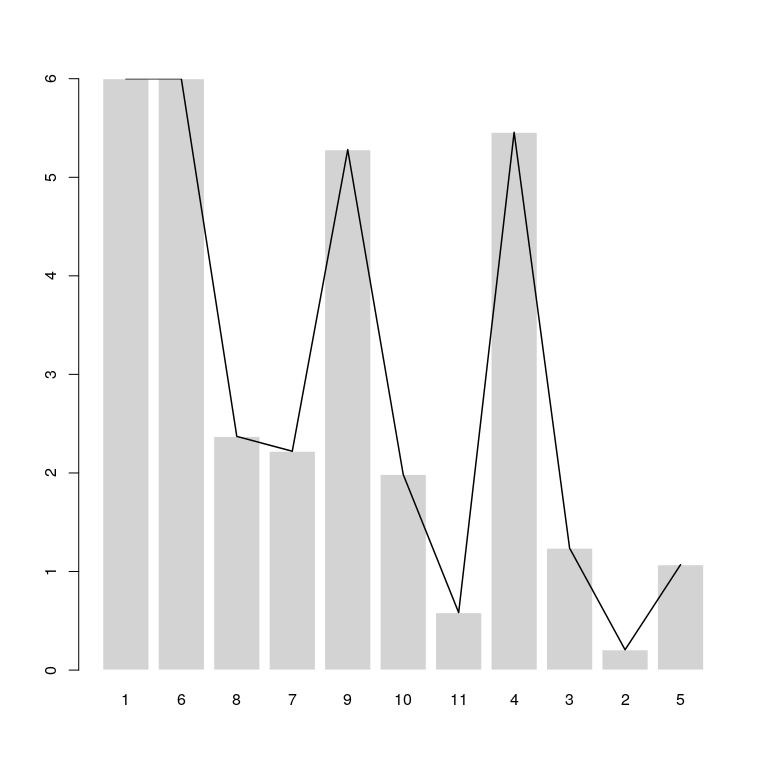epsilon
Is another OPTICS parameter (see there for more) and governs up to which distance we consider points to be neighbours in a cluster. This can be used in two ways: Either to make the OPTICS runtime quicker, which can be beneficial with a lot of points and, more importantly, to designate points as noise if the density in their vicinity is not large enough (in the OC, a noise point gets assigned $$d_{max}$$ and they are ordered next to each other so their reachbility difference is zero). In our situation we have a point that is dedicated as noise (point 1) and in the setup before (at least three points per cluster) it would therefore be included as a possible point in cluster 2 where it is in the neighbourhood (density-reachable) of the observations 6, 7, 8. This would however make that cluster very non-dense, giving high reachability from 7 (the succesor in the ordering) to that point and overall decreasing the OC.
ores3c<-cordillera(x,minpts=3,epsilon=10,dmax=6,scale=FALSE)
ores3c
#>       raw    normed
#> 7.4385041 0.4685817
plot(ores3c)We might not want that because we know it’s an outlier or that there is noise in our data, so we reduce epsilon to 7 and the point no longer becomes “density-reachable” from the other points. Now the point is no longer in any cluster and this makes the individual clusters comparatively more dense, meaning the OC increases

ores3d<-cordillera(x,minpts=3,epsilon=7,dmax=6,scale=FALSE)
ores3d
#>       raw    normed
#> 8.1302853 0.5121598
plot(ores3d)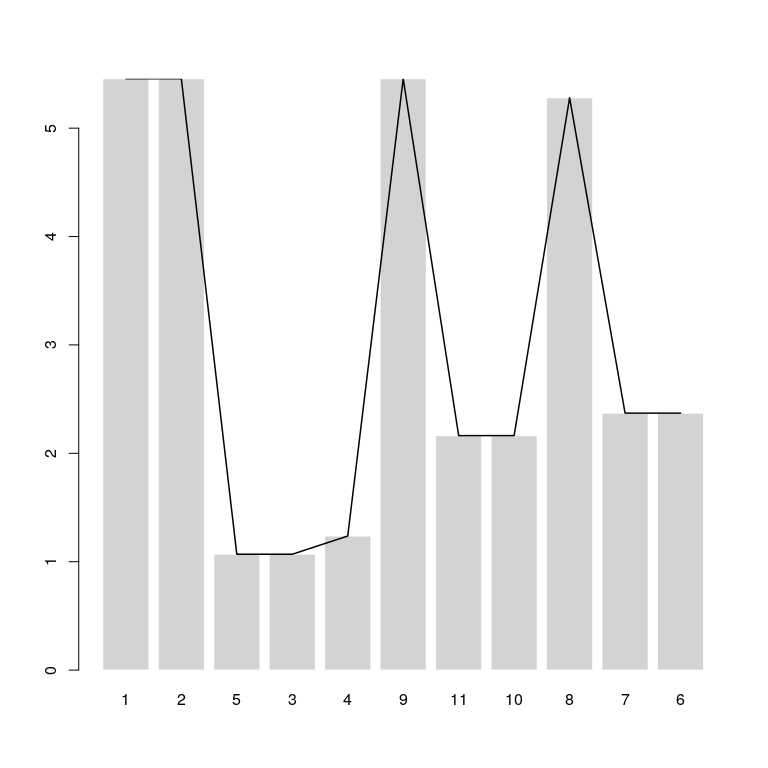Typically, though, this is a fickle parameter and it might be best to just leave it large enough to possibly include all points (that was OPTICS improvement over DBSCAN, to abstract from having to set $$\varepsilon$$. We also caution against setting epsilon too low, which can assign too many points as noise (optics reachability of $$\infty$$, or OC reachability of $$d_{max}$$), e.g.

ors<-optics(x,minPts=3,eps=5)
ors$reachdist #>  Inf Inf 1.068878 1.236932 1.068878 Inf 2.370654 3.008322 #>  Inf 2.163331 2.163331 dmax and rang These are parameters that allow for robustness in the OC. In the Cordillera, all reachabilities that are larger than dmax get winsorized to dmax (including points with a reachability of inf in OPTICS). For the normalization, dmax also plays a role as it is the maximum reference distance that needs to exist between neighbouring clusters in a perfect clusteredness situation. dmax should be larger than most reachabilities but smaller than any outlying reachability to not inflate the OC. For example in the situation with low clusteredness above (x3) ores3<-optics(x3,minPts=22,eps=10) plot(ores3)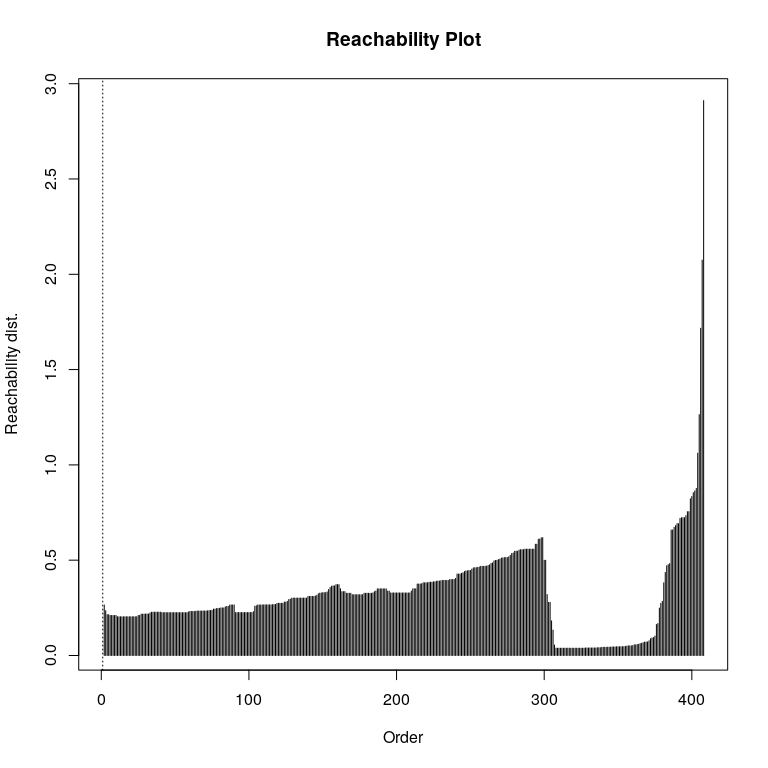We have a relatively large reachability (around 3) for the last point in the ordering. But the distribution of reachabilities is highly right-skewed and $$90\%$$ of the reachabilities are smaller than 0.54, when we look at the quantiles of the reachability distribution. quantile(ores3$reachdist[is.finite(ores3$reachdist)],p=seq(0,1,by=0.1)) #> 0% 10% 20% 30% 40% 50% 60% #> 0.03834048 0.04556087 0.20320570 0.22527262 0.24716444 0.29360350 0.32762482 #> 70% 80% 90% 100% #> 0.35811801 0.43940839 0.54724180 2.91112158 : The OC is by itself not robust to such outliers, so with the winsorization it allows to exclude the effect of these outliers. The default dmax is third quantile + 1.5 times the interquartile range, so on in our case here quantile(ores3$reachdist[is.finite(ores3$reachdist)],0.75)+1.5*IQR(ores3$reachdist[is.finite(ores3\$reachdist)])
#>       75%
#> 0.6397444

: this would be 0.64. Let’s compare the OC in these two cases

cres3e<-cordillera(x3,minpts=22,epsilon=10,dmax=2.9)
cres3e
#>      raw   normed
#> 2.858599 0.162052

cres3f<-cordillera(x3,minpts=22,epsilon=10,dmax=0.64)
cres3f
#>       raw    normed
#> 0.5163677 0.1326411

: where we see that the raw OC is much larger in the first case due to the outlier. This is a bit mitigated in the normed OC, but we still think that the robust version gives a more useful normalized version too. How to set dmax is of course up to the user, but it shouldn’t be too low either (e.g. set at the median because) this can result in too low a value (or even 0). The rang is the interval from any lower bound to any upper bound of the reachability and allows to fine tune the relative contribution for the OC if we want to measure the ups and downs not against zero, but against any other lower/upper bound for the reachabilities (e.g., the minimum and maximum reachability). We don’t expect users to use this parameter, though, and it’s default is (0,dmax). If it is given however and the maximum doesn’t agree with dmax, dmax is overridden, so use this with extreme caution.

q
Is a parameter relating to the OC and gives the $$L_p$$-space for the norm that is used for the OC. The most important values are q=1 where the sum of absolute reachability differences are taken over the ordering and q=2 where the Euclidean norm is used, so the square root of the sum of squared reachability differences over the ordering. For consistency, we believe q=1 is a good idea if the reachabilities are calculated from a matrix of Manhattan distances q=2 (the default) when calculated from a Euclidean distance matrix.
distmeth
Allows to calculate a distance matrix for the $$X$$ argument if it is not already a distance matrix. It supports all distances of stats::dist. Default is “euclidean”.
scale
this allows to scale the $$X$$ argument before calculating the cordillera. scale=FALSE or scale=0 takes $$X$$ as it is. If scale=TRUE or 1, standardisation is to mean=0 and sd=1 for each columns (not recommended as it distorts the $$X$$). If scale=2, no centering is applied and scaling of each column is done with the root mean square of each column. If scale=3, no centering is applied and scaling of all columns is done as X/max(standard deviation(allcolumns)). If scale=4, no centering is applied and scaling of all columns is done as X/max(rmsq(allcolumns)). Default is scale=FALSE. The scaling options are typically best used if we want to compare representations of the same data that can have different scales and where we are only interested in relative distances between points, as in most dimensions reduction methods.